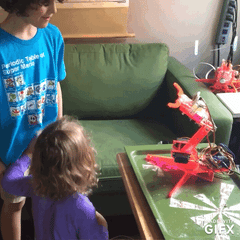# gridEye mimicArm Example

### gridEye mimicArm ExampleUsing the gridEye sensor we can detect the hottest point in the robots field of vision, which is great for detecting body heat! Using this example the mimicArm robot arm will follow you around the room. Or follow your brother down to the floor when you push him over.

Commands Used

gridEyeActivate();

robotActivate();

gridEyeRowMax();

gridEyeColMax();

robotJog(x,y);

robotMove(x,y);

if(conditional)

Cut and paste code:

/*
This example uses the GridEye sensor to follow the hottest object in the field of view, usually a person.
*/

#include <robotInputs.h>  //includes GridEye commands
#include <robot.h>        //Includes commands to move the robot

float pixelTable;  //the GridEye is an 8x8 array

void setup() {

gridEyeActivate();     //begin communication with the gridEye sensor
robotActivate();       //begin communication with the robot
//lets move the robot to a nice starting position
robotMove(1, 127);
robotMove(2, 160);
robotMove(3, 127);

}

void loop() {
robotMode(Arduino);  //remind the robot that it's taking commands from the Arduino
//These ints will hold the location of the hotest point in the array
static int moveCh3;
static int moveCh1;

//find highest temperature pixel
gridEyeRefresh();  //reading the gridEye sensor takes about 35 mS every time, so the functions only update every 70 mS to speed things up. gridEyeRefresh forces new values.
int highestTempCol = gridEyeColMax();  //find the highest temp 8x8 column

int highestTempRow = gridEyeRowMax();  //find the highest temp 8x8 row

moveCh3 = -1 * (highestTempRow - 4) * 1;
moveCh1 = (highestTempCol - 4) * 1;
//Serial.print(moveCh1); Serial.print(" "); Serial.println(robotPosition(1));
//Serial.print(moveCh3); Serial.print(" "); Serial.println(robotPosition(3));
//Serial.print("duration ");
//Serial.println(millis() - timer);

//if (!(robotPosition(1) >= 235 && moveCh1 > 1))robotJog(1, moveCh1);
//if (!(robotPosition(3) >= 254 && moveCh3 > 1))robotJog(3, moveCh3);

if(moveCh1) robotJog(1, moveCh1);  //theres no point in sending a new position if we're already in the center, so only move if moveCh1 != 0
if(moveCh3) robotJog(3, moveCh3);  //theres no point in sending a new position if we're already in the center, so only move if moveCh3 != 0
robotMove(2, 150 + (robotPosition(3) / 5));  //move Ch2 to make the robot look a little more lifelike and to reduce stress on the servo (the commutators get hot in one position)

}